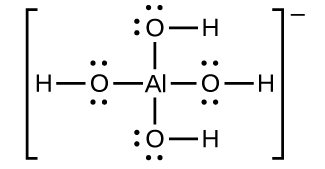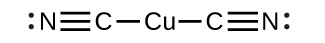# 15.2 Lewis acids and bases  (Page 2/12)

 Page 2 / 12The equations for the dissolution are:

$\text{Al}{\left(\text{OH}\right)}_{3}\left(s\right)\phantom{\rule{0.2em}{0ex}}⟶\phantom{\rule{0.2em}{0ex}}{\text{Al}}^{\text{3+}}\left(aq\right)+3{\text{OH}}^{\text{−}}\left(aq\right)$
${\text{Al}}^{\text{3+}}\left(aq\right)+4{\text{OH}}^{\text{−}}\left(aq\right)\phantom{\rule{0.2em}{0ex}}⟶\phantom{\rule{0.2em}{0ex}}\text{Al}{\left(\text{OH}\right)}_{4}{}^{\text{−}}\left(aq\right)$
$\text{Net:}\phantom{\rule{0.2em}{0ex}}\text{Al}{\left(\text{OH}\right)}_{3}\left(s\right)+{\text{OH}}^{\text{−}}\left(aq\right)\phantom{\rule{0.2em}{0ex}}⟶\phantom{\rule{0.2em}{0ex}}\text{Al}{\left(\text{OH}\right)}_{4}{}^{\text{−}}\left(aq\right)$

Mercury(II) sulfide dissolves in a solution of sodium sulfide because HgS reacts with the S 2– ion:

$\text{HgS}\left(s\right)\phantom{\rule{0.2em}{0ex}}⟶\phantom{\rule{0.2em}{0ex}}{\text{Hg}}^{\text{2+}}\left(aq\right)+{\text{S}}^{\text{2−}}\left(aq\right)$
${\text{Hg}}^{\text{2+}}\left(aq\right)+2{\text{S}}^{\text{2−}}\left(aq\right)\phantom{\rule{0.2em}{0ex}}⟶\phantom{\rule{0.2em}{0ex}}{\text{HgS}}_{2}{}^{\text{2−}}\left(aq\right)$
$\text{Net:}\phantom{\rule{0.2em}{0ex}}\text{HgS}\left(s\right)+{\text{S}}^{\text{2−}}\left(aq\right)\phantom{\rule{0.2em}{0ex}}⟶\phantom{\rule{0.2em}{0ex}}{\text{HgS}}_{2}{}^{\text{2−}}\left(aq\right)$

A complex ion consists of a central atom, typically a transition metal cation, surrounded by ions, or molecules called ligands . These ligands can be neutral molecules like H 2 O or NH 3 , or ions such as CN or OH . Often, the ligands act as Lewis bases, donating a pair of electrons to the central atom. The ligands aggregate themselves around the central atom, creating a new ion with a charge equal to the sum of the charges and, most often, a transitional metal ion. This more complex arrangement is why the resulting ion is called a complex ion . The complex ion formed in these reactions cannot be predicted; it must be determined experimentally. The types of bonds formed in complex ions are called coordinate covalent bonds, as electrons from the ligands are being shared with the central atom. Because of this, complex ions are sometimes referred to as coordination complexes. This will be studied further in upcoming chapters.

The equilibrium constant for the reaction of the components of a complex ion to form the complex ion in solution is called a formation constant ( K f ) (sometimes called a stability constant). For example, the complex ion $\text{Cu}{\left(\text{CN}\right)}_{2}{}^{\text{−}}$ is shown here:It forms by the reaction:

${\text{Cu}}^{\text{+}}\left(aq\right)+2{\text{CN}}^{\text{−}}\left(aq\right)⇌\text{Cu}{\left(\text{CN}\right)}_{2}{}^{\text{−}}\left(aq\right)$

At equilibrium:

${K}_{\text{f}}\phantom{\rule{0.2em}{0ex}}=\phantom{\rule{0.2em}{0ex}}Q=\frac{\left[\text{Cu}{\left(\text{CN}\right)}_{2}{}^{\text{−}}\right]}{\left[{\text{Cu}}^{+}\right]{\left[{\text{CN}}^{\text{−}}\right]}^{2}}$

The inverse of the formation constant is the dissociation constant ( K d ) , the equilibrium constant for the decomposition of a complex ion into its components in solution. We will work with dissociation constants further in the exercises for this section. Appendix K and [link] are tables of formation constants. In general, the larger the formation constant, the more stable the complex; however, as in the case of K sp values, the stoichiometry of the compound must be considered.

Common Complex Ions by Decreasing Formulation Constants
Substance K f at 25 °C
${\left[\text{Cd}{\left(\text{CN}\right)}_{4}\right]}^{\text{2−}}$ 3 $×$ 10 18
$\text{Ag}{\left({\text{NH}}_{3}\right)}_{2}{}^{+}$ 1.7 $×$ 10 7
${\left[{\text{AlF}}_{6}\right]}^{\text{3−}}$ 7 $×$ 10 19

As an example of dissolution by complex ion formation, let us consider what happens when we add aqueous ammonia to a mixture of silver chloride and water. Silver chloride dissolves slightly in water, giving a small concentration of Ag + ([Ag + ] = 1.3 $×$ 10 –5 M ):

$\text{AgCl}\left(s\right)⇌{\text{Ag}}^{\text{+}}\left(aq\right)+{\text{Cl}}^{\text{−}}\left(aq\right)$

However, if NH 3 is present in the water, the complex ion, $\text{Ag}{\left({\text{NH}}_{3}\right)}_{2}{}^{+},$ can form according to the equation:

${\text{Ag}}^{\text{+}}\left(aq\right)+2{\text{NH}}_{3}\left(aq\right)⇌\text{Ag}{\left({\text{NH}}_{3}\right)}_{2}{}^{\text{+}}\left(aq\right)$

with

${K}_{\text{f}}=\frac{\left[\text{Ag}{\left({\text{NH}}_{3}\right)}_{2}{}^{+}\right]}{\left[{\text{Ag}}^{+}\right]{\left[{\text{NH}}_{3}\right]}^{2}}\phantom{\rule{0.2em}{0ex}}=1.7\phantom{\rule{0.2em}{0ex}}×\phantom{\rule{0.2em}{0ex}}{10}^{7}$

The large size of this formation constant indicates that most of the free silver ions produced by the dissolution of AgCl combine with NH 3 to form $\text{Ag}{\left({\text{NH}}_{3}\right)}_{2}{}^{+}.$ As a consequence, the concentration of silver ions, [Ag + ], is reduced, and the reaction quotient for the dissolution of silver chloride, [Ag + ][Cl ], falls below the solubility product of AgCl:

$Q\phantom{\rule{0.2em}{0ex}}=\phantom{\rule{0.2em}{0ex}}\left[{\text{Ag}}^{+}\right]\left[{\text{Cl}}^{\text{−}}\right]\phantom{\rule{0.2em}{0ex}}<\phantom{\rule{0.2em}{0ex}}{K}_{\text{sp}}$

More silver chloride then dissolves. If the concentration of ammonia is great enough, all of the silver chloride dissolves.

what are oxidation numbers
pls what is electrolysis
Electrolysis is the process by which ionic substances are decomposed (broken down) into simpler substances when an electric current is passed through them. ... Electricity is the flow of electrons or ions. For electrolysis to work, the compound must contain ions.
AZEEZ
thanks
Idowu
what is the basicity of an atom
basicity is the number of replaceable Hydrogen atoms in a Molecule. in H2SO4, the basicity is 2. in Hcl, the basicity is 1
Inemesit
how to solve oxidation number
mention some examples of ester
do you mean ether?
Megan
what do converging lines on a mass Spectra represent
would I do to help me know this topic ?
Bulus
oi
Amargo
what the physic?
who is albert heistein?
Bassidi
similarities between elements in the same group and period
what is the ratio of hydrogen to oxulygen in carbohydrates
bunubyyvyhinuvgtvbjnjnygtcrc
yvcrzezalakhhehuzhbshsunakakoaak
what is poh and ph
please what is the chemical configuration of sodium
Sharon
2.8.1
david
1s²2s²2p⁶3s¹
Haile
2, 6, 2, 1
Salman
1s2, 2s2, 2px2, 2py2, 2pz2, 3s1
Justice
1s2,2s2,2py2,2
Maryify
1s2,2s2,2p6,
Francis
1s2,2s2,2px2,2py2,2pz2,3s1
Nnyila
what is criteria purity
cathode is a negative ion why is it that u said is negative
cathode is a negative electrode while cation is a positive ion. cation move towards cathode plate.
king
CH3COOH +NaOH ,complete the equation
compare and contrast the electrical conductivity of HCl and CH3cooH
The must be in dissolved in water (aqueous). Electrical conductivity is measured in Siemens (s). HCl (aq) has higher conductivity, as it fully ionises (small portion of CH3COOH (aq) ionises) when dissolved in water. Thus, more free ions to carry charge.
Abdelkarim
HCl being an strong acid will fully ionize in water thus producing more mobile ions for electrical conduction than the carboxylic acid
Valentine
differiante between a weak and a strong acid
david
how can I tell when an acid is weak or Strong
Amarachi
an aqueous solution of copper sulphate was electrolysed between graphite electrodes. state what was observed at the cathode
write the equation for the reaction that took place at the anode
Bakanya
what is enthalpy of combustion
Bakanya
Enthalpy change of combustion: It is the enthalpy change when 1 mole of substance is combusted with excess oxygen under standard conditions. Elements are in their standard states. Conditions: pressure = 1 atm Temperature =25°C
Abdelkarim
Observation at Cathode: Cu metal deposit (pink/red solid).
Abdelkarim
Equation at Anode: (SO4)^2- + 4H^+ + 2e^- __> SO2 + 2H2O
Abdelkarim
Equation : CuSO4 -> Cu^2+ + SO4^2- equation at katode: 2Cu^2+ + 4e -> 2Cu equation at anode: 2H2O -> 4H+ + O2 +4e at the anode which reacts is water because SO4 ^ 2- cannot be electrolyzed in the anode
NikenByByBy Steve GibbsBy OpenStaxBy Kimberly NicholsBy Rohini AjayBy Madison ChristianBy Vongkol HENGBy Megan EarhartBy Brianna BeckBy Madison ChristianBy